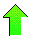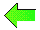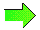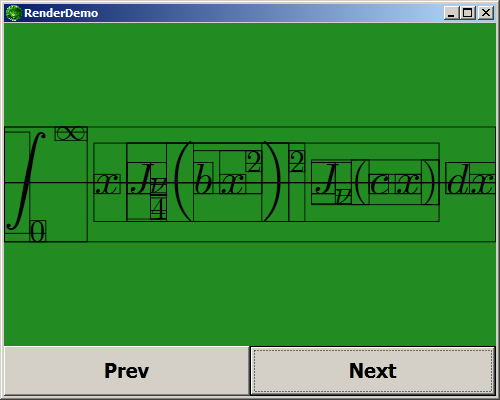Manual - IntroductionContents News View

 Introduction - FeaturesFeatures1.  High-level, Interpreted, Interactive, Symbolic Language 2.  .NET Windows Programming Language (.NET 4, WPF, XAML) 3.  Computer Algebra System 4.  Syntax and Semantics Strongly Resembling Lisp 5.  Risch's Algorithm (Calculus) 6.  Cherry's Algorithm (Calculus) 7.  Elliptic Integrals (Calculus) 8.  Hypergeometric Integrals (Calculus) 9.  Multivariate Polynomial Factorization, Gcd 10.  Int, Solve, Series, Limit, Sum, Resultant 11.  Matrix Algebra (RowReduce, MatrixInverse, NullSpace) 12.  Grobner Bases, Buchberger's Algorithm 13.  Linear Programming 14.  Number Theory (DiscreteLog, InverseEulerPhi, JacobiSymbol, PrimitiveRoot) 15.  Arbitrary Precision Integer's, Float's, and Complex's 16.  Mathematical Constants (_Pi, _E, _Catalan, _EulerGamma, _I, _Infinity) 17.  Elementary Functions 18.  Special Functions (Elliptic, Gamma, Hypergeometric, RiemannZeta) 19.  Numerical Analysis (Int, Solve) 20.  Symbolic and Numeric HyperF 21.  Symbolic and Numeric MeijerG 22.  Statistics (ChiSquare, StudentT, Regression) 23.  Mathematical Plotting 24.  Mathematical Publishing 25.  Good for Experimental AI Work